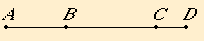# Proposition 82

To a minor straight line only one straight line can be annexed which is incommensurable in square with the whole and which makes, with the whole, the sum of squares on them rational but twice the rectangle contained by them medial.
X.76

Let AB be the minor straight line, and let BC be an annex to AB. Then AC and CB are straight lines incommensurable in square which make the sum of the squares on them rational, but twice the rectangle contained by them medial.

I say that no other straight line can be annexed to AB fulfilling the same conditions.X.76

If possible, let BD be so annexed. Then AD and DB are both straight lines incommensurable in square which fulfill the aforesaid conditions.

X.26

Now, since the excess of the sum of the squares on AD and DB over the sum of the squares on AC and CB is also the excess of twice the rectangle AD by DB over twice the rectangle AC by CB, while the sum of the squares on AD and DB exceed the sum of the squares on AC and CB by a rational area, for both are rational, therefore twice the rectangle AD by DB also exceeds twice the rectangle AC by CB by a rational area, which is impossible, for both are medial.

Therefore, to a minor straight line only one straight line can be annexed which is incommensurable in square with the whole and which makes, with the whole, the sum of squares on them rational but twice the rectangle contained by them medial.

Q.E.D.

## Guide

This proposition is not used in the rest of the Elements.